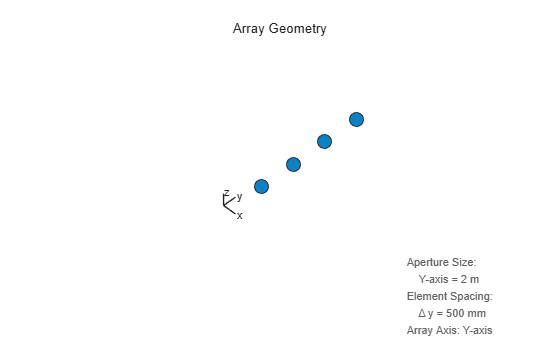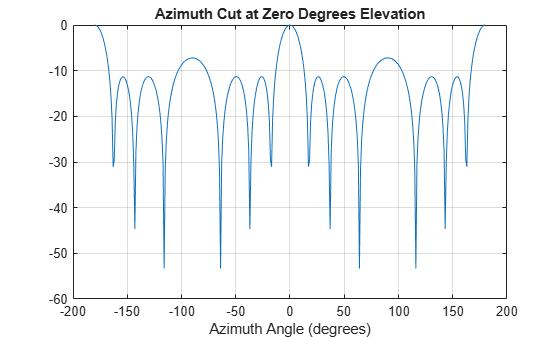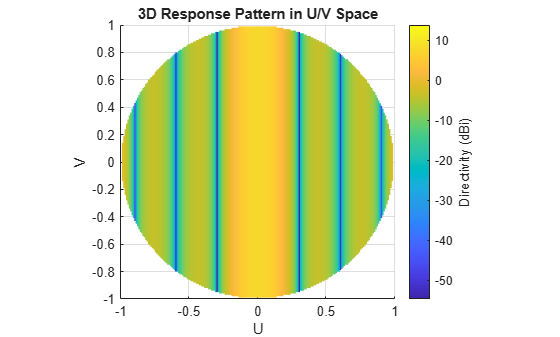Main Content

## Uniform Linear Array

### Support for Uniform Linear Arrays

The uniform linear array (ULA) arranges identical sensor elements along a line in space with uniform spacing. You can design a ULA with `phased.ULA`. When you use this object, you must specify these aspects of the array:

• Sensor elements of the array

• Spacing between array elements

• Number of elements in the array

### Positions of ULA Array Elements

Create and view a ULA having four isotropic antenna elements separated by 0.5 meters

```array = phased.ULA('NumElements',4,'ElementSpacing',0.5); viewArray(array);```You can return the coordinates of the array sensor elements in the form `[x;y;z]` by using the `getElementPosition` method. See Rectangular Coordinates for toolbox conventions.

`sensorpos = getElementPosition(array);`

`sensorpos` is a 3-by-4 matrix with each column representing the position of a sensor element. Note that the y-axis is the array axis. The positive x-axis is the array look direction (0 degrees broadside). The elements are symmetric with the respect to the phase center of the array.

### ULA Array Elements

The default element for a ULA is the `phased.IsotropicAntennaElement` object. You can specify a different element using the `Element` property.

### Array Element Responses

Obtain the responses of the elements of a 4-element ULA array at 1 GHz.

Note: This example runs only in R2016b or later. If you are using an earlier release, replace each call to the function with the equivalent `step` syntax. For example, replace `myObject(x)` with `step(myObject,x)`.

Specify isotopic antennas for the array elements. Then, specify a 4-element ULA. Obtain the response by executing the System object™.

```antenna = phased.IsotropicAntennaElement(... 'FrequencyRange',[3e8 1e9]); array = phased.ULA('NumElements',4,'ElementSpacing',0.5,... 'Element',antenna); freq = 1e9; azangles = -180:180; response = array(freq,azangles);```

`response` is a 4-by-361 matrix where each column contains the responses at each azimuth angle. Matrix rows correspond to the four elements. Because the elements of the ULA are isotropic antennas, `response` is a matrix of ones.

### Signal Delay Between Array Elements

This example computes the delay between elements of a 4-element ULA using the `phased.ElementDelay` System object™. Assume that the incident waveform satisfies the far-field condition. The delays are computed with respect to the phase center of the array. By default, `phased.ElementDelay` assumes that the incident waveform is an electromagnetic wave propagating at the speed of light.

Note: This example runs only in R2016b or later. If you are using an earlier release, replace each call to the function with the equivalent `step` syntax. For example, replace `myObject(x)` with `step(myObject,x)`.

Construct 4-element ULA using value-only syntax and compute the delay for a signal incident on the array from -90° azimuth and 0° elevation. Delay units are in seconds.

```array = phased.ULA(4); delay = phased.ElementDelay('SensorArray',array); tau = delay([-90;0])```
```tau = 4×1 10-8 × -0.2502 -0.0834 0.0834 0.2502 ```

`tau` is a 4-by-1 vector of delays with respect to the phase center of the array, which is the origin of the local coordinate system (0;0;0). See Global and Local Coordinate Systems for a description of global and local coordinate systems. Negative delays indicate that the signal arrives at an element before reaching the phase center of the array. Because the waveform arrives from an azimuth angle of -90°, the signal arrives at the first and second elements of the ULA before it reaches the phase center resulting in negative delays for these elements.

If the signal is incident on the array at 0° broadside from a far-field source, the signal illuminates all elements of the array simultaneously resulting in zero delay.

`tau = delay([0;0])`
```tau = 4×1 0 0 0 0 ```

If the incident signal is an acoustic pressure waveform propagating at the speed of sound, you can calculate the element delays by setting the `PropagationSpeed` property to 340 m/s. This value is a typical speed of sound at sea level.

```delay = phased.ElementDelay('SensorArray',array,... 'PropagationSpeed',340); tau = delay([90;0])```
```tau = 4×1 0.0022 0.0007 -0.0007 -0.0022 ```

### Steering Vector

The steering vector represents the relative phase shifts for the incident far-field waveform across the array elements. You can determine these phase shifts with the `phased.SteeringVector` object.

For a single carrier frequency, the steering vector for a ULA consisting of N elements is:

`$\left(\begin{array}{c}{e}^{-j2\pi f{\tau }_{1}}\\ {e}^{-j2\pi f{\tau }_{2}}\\ {e}^{-j2\pi f{\tau }_{3}}\\ .\\ .\\ .\\ {e}^{-j2\pi f{\tau }_{N}}\end{array}\right)$`

where τn denotes the time delay relative to the array phase center at the n-th array element.

#### Compute ULA Steering Vector

Compute the steering vector for a 4-element ULA at an operating frequency of 1 GHz. Assume that the waveform is incident on the array from 45° azimuth and 10° elevation.

Note: This example runs only in R2016b or later. If you are using an earlier release, replace each call to the function with the equivalent `step` syntax. For example, replace `myObject(x)` with `step(myObject,x)`.

```fc = 1e9; array = phased.ULA(4); steervec = phased.SteeringVector('SensorArray',array); sv = steervec(fc,[45;10])```
```sv = 4×1 complex -0.0495 + 0.9988i -0.8742 + 0.4856i -0.8742 - 0.4856i -0.0495 - 0.9988i ```

You can also compute the steering vector with the following equivalent code.

```delay = phased.ElementDelay('SensorArray',array); tau = delay([45;10]); exp(-1i*2*pi*fc*tau)```
```ans = 4×1 complex -0.0495 + 0.9988i -0.8742 + 0.4856i -0.8742 - 0.4856i -0.0495 - 0.9988i ```

### Array Response

To obtain the array response, which is a weighted-combination of the steering vector elements for each incident angle, use the `phased.ArrayResponse` System object™.

#### ULA Array Response

Construct a four-element ULA with elements spaced at 0.25 m. Obtain the array magnitude response (absolute value of the complex-valued array response) for azimuth angles (-180:180) at 1 GHz. Then, plot the normalized magnitude response in dB.

Note: This example runs only in R2016b or later. If you are using an earlier release, replace each call to the function with the equivalent `step` syntax. For example, replace `myObject(x)` with `step(myObject,x)`.

```fc = 1e9; array = phased.ULA('NumElements',4,'ElementSpacing',0.25); azangles = -180:180; response = phased.ArrayResponse('SensorArray',array); resp = abs(response(fc,azangles)); plot(azangles,mag2db((resp/max(resp)))) grid on title('Azimuth Cut at Zero Degrees Elevation') xlabel('Azimuth Angle (degrees)')```Visualize the array response using the `pattern` method. Create a 3-D plot of the response in UV space; other plotting options are available.

```pattern(array,fc,[-1:.01:1],[-1:.01:1],'CoordinateSystem','uv',... 'PropagationSpeed',physconst('Lightspeed'))```### Reception of Plane Wave Across Array

You can simulate the effects of phase shifts across your array using the `collectPlaneWave` method of any array System object.

The `collectPlaneWave` method modulates input signals by the element of the steering vector corresponding to an array element. Stated differently, `collectPlaneWave` accounts for phase shifts across elements in the array based on the angle of arrival. However, `collectPlaneWave` does not account for the response of individual elements in the array.

#### Plane-Wave Reception Across ULA

Simulate the reception of a 100-Hz sine wave modulated by a carrier frequency of 1 GHz at a 4-element ULA. Assume the angle of arrival of the signal is (-90;0).

Note: This example runs only in R2016b or later. If you are using an earlier release, replace each call to the function with the equivalent `step` syntax. For example, replace `myObject(x)` with `step(myObject,x)`.

```array = phased.ULA(4); t = unigrid(0,0.001,0.01,'[)'); x = cos(2*pi*100*t)'; y = collectPlaneWave(array,x,[-90;0],1e9,physconst('LightSpeed'));```

The preceding code is equivalent to the following.

```steervec = phased.SteeringVector('SensorArray',array); sv = steervec(1e9,[-90;0]); y1 = x*sv.';```

## Support

#### Exploring Hybrid Beamforming Architectures for 5G Systems

Download white paper